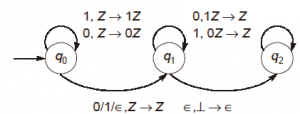Related Articles
GATE | GATE-CS-2015 (Set 1) | Question 60
• Last Updated : 20 Feb, 2015

Consider the NPDA 〈Q = {q0, q1, q2}, Σ = {0, 1}, Γ = {0, 1, ⊥}, δ, q0, ⊥, F = {q2}〉, where (as per usual convention) Q is the set of states, Σ is the input alphabet, Γ is stack alphabet, δ is the state transition function, q0 is the initial state, ⊥ is the initial stack symbol, and F is the set of accepting states, The state transition is as follows:Which one of the following sequences must follow the string 101100 so that the overall string is accepted by the automaton?
(A) 10110
(B) 10010
(C) 01010
(D) 01001

Explanation: In q0 state for ‘1’, a ‘1’ is pushed and for a ‘0’ a ‘0’ is pushed. In q1 state, for a ‘0’ a ‘1’ is popped and for a ‘1’ a ‘0’ is popped. So, the given PDA is accepting all strings of of the form x0x’r or x1x’r or xx’r, where x’r is the reverse of the 1’s complement of x.

The given string 101100 has 6 letters and we are given 5 letter strings. So, x0 is done, with x = 10110. So, x’r = (01001)r = 10010.

Hence option B is correct.

Quiz of this QuestionMy Personal Notes arrow_drop_up
Recommended Articles
Page :